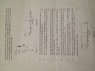# Solving Transient Response RL Circuit with Switch

• van123
In summary, the question is asking if when the switch is closed, there is no current flowing through the right side of the circuit (the resistor and diode). The answer is no, because the current through the diode increases when the switch is closed.

## Homework Statement

I have a circuit containing an inductor, 2 resistors and diode in series however there is also a switch.

## The Attempt at a Solution

I thought that when the switch is closed there would be no current flowing through the right side of the circuit (resistor and diode) and therefore for part a) the time constant is L/R=10/20=0.5ms and steady state inductor current is just V/R=0.5A.
However, this can't be the case in order to do the show that question in part C), so I was wondering if someone could just explain the circuit to me when the switch is open and closed. Thank you!

#### Attachments

•IMG_0335.jpeg
47.5 KB · Views: 509
What, in toto, is the question?
Never mind, I got the picture straightened out. Stand by ...

Last edited:
How it works: at t=0 swirch S is closed and the current thru the inductor increases. At t = 0.5ms S opens and the inductor current continues thru the diode until iL = 0 at t = TZ. The current stays at zero until S closes again, at which point the cycle repeats.

Part (c) is misleadingly stated. The current thru the diode is -10 mA only if the switch stays open for a long time.

(d) is an important computation. You will need it for part (e).

Part (f) is more challenging because the current thru the diode does not ever equal zero.

Reattempt

Thank you rude man so much for explaining, i have tried to answer the entire question again from what I (hope) i now understand. If you have time could you perhaps see my answers and tell me if I'm still not getting it?
Thanks again!

van123 said:
Thank you rude man so much for explaining, i have tried to answer the entire question again from what I (hope) i now understand. If you have time could you perhaps see my answers and tell me if I'm still not getting it?
Thanks again!